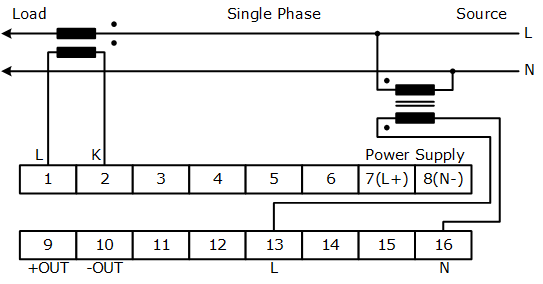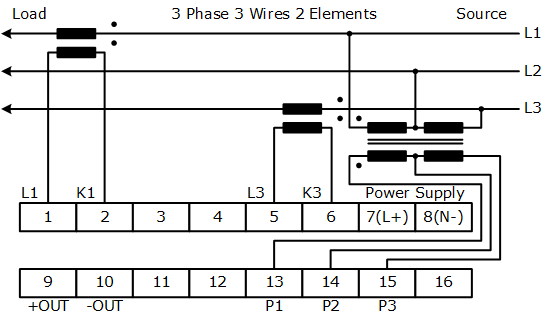# WAT95: Watt Transducer- High accuracy - Long term stability - Time-Division Multiplication principle

Watt Transducers WAT95 are used to convert single or three phaseunbalance load active power into a proportional load independent DC. Output. Based fully on electronic Time-Division Multiplication measuring principle, the true power of a network with any power factor range are linearly produced by Watt Transducer output.Calibration watt of rated output Connection V=110V V=220V V=380V I=1A I=5A I=1A I=5A I=1A I=5A Single Phase 100W 500W 200W 1000W - - 3phase,3wire 200W 1000W 400W 2000W 600W 3000W 3phase,4wire 200W 1000W 400W 2000W 600W 3000W
 Note. V= Line voltage Equation to calculated measuring range Measuring range = Calibration watt x PT ratio x CT ratio Example 3phase,3wire, PT 220V/110V, CT 250/5A, Calibration watt = 1000W Measuring range = 1000 x 220/110 x 250/5 = 100 KW.

Specifications

Input

Number of Channel:

3 Channels

Input Type:

Current, Voltage (1 phase,3 phase 3 wire, 3 phase 4 wire)

Input Range:

Current (1, 5 A), Accuracy Class: 0.5

Voltage (110, 220, 380 VAC (Line voltage for 3 phase)

Power Consumption:

< 0.2 VA for input current, < 0.4 VA for voltage input

Frequency:

50 Hz.

Analog Output

Number of Channel:

1 Channel

Output Type:

Current, Voltage

Output Range:

Current Max 0 to 1 mA (Load Resistance 10 KΩ)

Current Max 4 to 20 mA (Load Resistance 600 Ω)

Voltage Min 0 to 10 mVDC (Load Resistance 500 Ω)

Voltage Min 0 to 100 mVDC (Load Resistance 500 Ω)

Voltage Min 0 to 1 VDC (Load Resistance 500 Ω)

Voltage Min 0 to 5 VDC (Load Resistance 1 KΩ)

Voltage Min 1 to 5 VDC (Load Resistance 1 KΩ)

Voltage Min 0 to 10 VDC (Load Resistance 2 KΩ)

Linearity:

< ± 0.5 % of span

Power Requirements

Power Supply:

110, 220 VAC

Environmental Limits

Operating Temperature:

0 to 55 °C

Operating Humidity:

5 to 95 %RH

Storage Temperature:

0 to 70 °C

Physical Characteristics

Dimension:

W132 x H132 x D162 mm.

Mounting:

Wall Mount

Wiring:

Screw Terminals

Protection:

IP20

Warranty

Warranty Period:

5 year

Ordering
 Ordering Information: Specify Connection, Input voltage, Input current, Output, Power supply Example WAT95/3 phase 4 wire/380 VAC/5A/4-20 mA/220 VAC
Packagelist

Package Checklist

1. WAT95WAT95 Specification.pdf